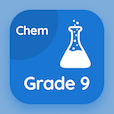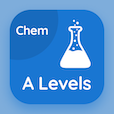SAT Online Courses

SAT Chemistry MCQs

SAT Chemistry MCQ PDF - Topics

# Introduction MCQ Quiz Online

Learn Introduction Multiple Choice Questions (MCQ), Introduction quiz answers PDF to study sat chemistry online course for sat chemistry classes. Oxidation-Reduction Multiple Choice Questions and Answers (MCQs), Introduction quiz questions for college entrance exams. "Introduction MCQ" PDF Book: oxidation-reduction test prep for job placement test.

"To maintain the electrical neutrality, we use" MCQ PDF: introduction with choices acid bridge, base bridge, salt bridge, and all of above for college entrance exams. Study introduction quiz questions for merit scholarship test and certificate programs for employment assessment test.

## MCQs on Introduction Quiz

MCQ: To maintain the electrical neutrality, we use

Acid bridge
Base bridge
Salt bridge
All of above

MCQ: The conversion of chemical energy into electrical energy requires

Electrolytic cell
Galvanic cell
Voltaic cell
Both b and c

MCQ: The combustion of carbon in oxygen is an example of

Endothermic reaction
Exothermic reaction
Displacement reaction
Neutralization reaction

MCQ: Reduction or oxidation potential of standard hydrogen electrode is

0.0 volt
1.0 volt
0.8 volt
1.8 volt

MCQ: Human blood has a pH of

8.35
9.35
7.35
6.35

### More Topics from SAT Chemistry Course